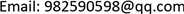﻿ 亚纯函数涉及分担值集的一个结果 A Result of Meromorphic Functions Involving Sharing Value Sets

Pure Mathematics
Vol. 10  No. 01 ( 2020 ), Article ID: 34039 , 5 pages
10.12677/PM.2020.101007

A Result of Meromorphic Functions Involving Sharing Value Sets

Ming Lai, Ronghui Li

School of Mathematics, Yunnan Normal University, Kunming YunnanReceived: Dec. 20th, 2019; accepted: Jan. 10th, 2020; published: Jan. 19th, 2020ABSTRACT

This paper proves that if two nonconstant meromorphic functions f and g satisfy $\Theta \left(\infty ,f\right)>\frac{1}{2}$,$\Theta \left(\infty ,g\right)>\frac{1}{2}$, there must exist a set $S\subset C$ with 6 elements, and if $E\left(S,f\right)=E\left(S,g\right)$, there is $T\left(r,f\right)\sim T\left(r,g\right)$.

Keywords:Meromorphic Function, Shared Value Set, Characteristic Function1. 引言及主要结果

$f\left(z\right)$ 为非常数亚纯函数，S为一个非空集合。令 $E\left(S,f\right)=\underset{a\in S}{\cup }\left\{z|f\left(z\right)-a=0\right\}$，这里m重零点在 $E\left(S,f\right)$ 中重复m次，则称 $E\left(S,f\right)$ 为f下S的原象集合。

1968年，F. Gross  研究了将公共值推广到公共值集的一个一般性的情况，如下所示：

1976年，F. Gross  提出了这样一个问题：

1993年，仪洪勋  对上述问题的结论进行了弱化并得到了如下结论：

2. 引理

(i) $g\left(z\right)$$f\left(z\right)$ 的分式线性变换；

(ii) $\left(1-o\left(1\right)\right)T\left(r\right)\le {N}_{2}\left(r,\frac{1}{f}\right)+{N}_{2}\left(r,\frac{1}{g}\right)+2\stackrel{¯}{N}\left(r,f\right)+2\stackrel{¯}{N}\left(r,g\right)+S\left(r,f\right)+S\left(r,g\right),$ (1)

$H=\left\{\frac{{f}^{″}}{{f}^{\prime }}-2\frac{{f}^{\prime }}{f-1}\right\}-\left\{\frac{{g}^{″}}{{g}^{\prime }}-2\frac{{g}^{\prime }}{g-1}\right\},$ (2)

$H\equiv 0$，则

$\frac{{f}^{″}}{{f}^{\prime }}-2\frac{{f}^{\prime }}{f-1}\equiv \frac{{g}^{″}}{{g}^{\prime }}-2\frac{{g}^{\prime }}{g-1},$ (3)

$H\overline{)\equiv }0$，则由对数导数引理知：

$m\left(r,H\right)=S\left(r,f\right)+S\left(r,g\right),$ (4)

$N\left(r,H\right)\le \stackrel{¯}{N}\left(r,f\right)+\stackrel{¯}{N}\left(r,g\right)+{\stackrel{¯}{N}}_{\left(2}\left(r,\frac{1}{f}\right)+{\stackrel{¯}{N}}_{\left(2}\left(r,\frac{1}{g}\right)+{\stackrel{¯}{N}}_{\text{0}}\left(r,\frac{1}{{f}^{\prime }}\right)+{\stackrel{¯}{N}}_{\text{0}}\left(r,\frac{1}{{g}^{\prime }}\right)+S\left(r,f\right)+S\left(r,g\right),$ (5)

$T\left(r,H\right)\le \stackrel{¯}{N}\left(r,f\right)+\stackrel{¯}{N}\left(r,g\right)+{\stackrel{¯}{N}}_{\left(2}\left(r,\frac{1}{f}\right)+{\stackrel{¯}{N}}_{\left(2}\left(r,\frac{1}{g}\right)+{\stackrel{¯}{N}}_{\text{0}}\left(r,\frac{1}{{f}^{\prime }}\right)+{\stackrel{¯}{N}}_{\text{0}}\left(r,\frac{1}{{g}^{\prime }}\right)+S\left(r,f\right)+S\left(r,g\right),$ (6)

$\begin{array}{c}{\stackrel{¯}{N}}_{1\right)}\left(r,\frac{1}{f-1}\right)={\stackrel{¯}{N}}_{1\right)}\left(r,\frac{1}{g-1}\right)\le N\left(r,\frac{1}{H}\right)\le T\left(r,H\right)+O\left(1\right)\\ \le \stackrel{¯}{N}\left(r,f\right)+\stackrel{¯}{N}\left(r,g\right)+{\stackrel{¯}{N}}_{\left(2}\left(r,\frac{1}{f}\right)+{\stackrel{¯}{N}}_{\left(2}\left(r,\frac{1}{g}\right)\\ \text{\hspace{0.17em}}\text{\hspace{0.17em}}+{\stackrel{¯}{N}}_{\text{0}}\left(r,\frac{1}{{f}^{\prime }}\right)+{\stackrel{¯}{N}}_{\text{0}}\left(r,\frac{1}{{g}^{\prime }}\right)+S\left(r,f\right)+S\left(r,g\right),\end{array}$ (7)

$\begin{array}{c}T\left(r,f\right)+T\left(r,g\right)\le \stackrel{¯}{N}\left(r,f\right)+\stackrel{¯}{N}\left(r,g\right)+\stackrel{¯}{N}\left(r,\frac{1}{f}\right)+\stackrel{¯}{N}\left(r,\frac{1}{g}\right)+\stackrel{¯}{N}\left(r,\frac{1}{f-1}\right)\\ \text{\hspace{0.17em}}\text{\hspace{0.17em}}+\stackrel{¯}{N}\left(r,\frac{1}{g-1}\right)-{\stackrel{¯}{N}}_{\text{0}}\left(r,\frac{1}{{f}^{\prime }}\right)-{\stackrel{¯}{N}}_{\text{0}}\left(r,\frac{1}{{g}^{\prime }}\right)+S\left(r,f\right)+S\left(r,g\right),\end{array}$ (8)

$\begin{array}{l}\stackrel{¯}{N}\left(r,\frac{1}{f-1}\right)+\stackrel{¯}{N}\left(r,\frac{1}{g-1}\right)=2\stackrel{¯}{N}\left(r,\frac{1}{f-1}\right)\le {\stackrel{¯}{N}}_{1\right)}\left(r,\frac{1}{f-1}\right)+N\left(r,\frac{1}{f-1}\right)\\ \le N\left(r,\frac{1}{f-1}\right)+\stackrel{¯}{N}\left(r,f\right)+\stackrel{¯}{N}\left(r,g\right)+{\stackrel{¯}{N}}_{\left(2}\left(r,\frac{1}{f}\right)+{\stackrel{¯}{N}}_{\left(2}\left(r,\frac{1}{g}\right)\\ \text{\hspace{0.17em}}\text{\hspace{0.17em}}+{\stackrel{¯}{N}}_{\text{0}}\left(r,\frac{1}{{f}^{\prime }}\right)+{\stackrel{¯}{N}}_{\text{0}}\left(r,\frac{1}{{g}^{\prime }}\right)+S\left(r,f\right)+S\left(r,g\right),\end{array}$ (9)

$T\left(r,f\right)+T\left(r,g\right)\le {N}_{2}\left(r,\frac{1}{f}\right)+{N}_{2}\left(r,\frac{1}{g}\right)+2\stackrel{¯}{N}\left(r,f\right)+2\stackrel{¯}{N}\left(r,g\right)+N\left(r,\frac{1}{f-1}\right)+S\left(r,f\right)+S\left(r,g\right),$ (10)

$N\left(r,\frac{1}{f-1}\right)\le T\left(r,f\right)+O\left(1\right),$ (11)

$N\left(r,\frac{1}{f-1}\right)=N\left(r,\frac{1}{g-1}\right)\le T\left(r,g\right)+O\left(1\right),$ (12)

$\left(1-o\left(1\right)\right)T\left(r\right)\le {N}_{2}\left(r,\frac{1}{f}\right)+{N}_{2}\left(r,\frac{1}{g}\right)+2\stackrel{¯}{N}\left(r,f\right)+2\stackrel{¯}{N}\left(r,g\right)+S\left(r,f\right)+S\left(r,g\right),$ (13)

$\left(r\notin E,r\to \infty ,mesE<+\infty \right)$

3. 定理1的证明

$P\left(w\right)={w}^{n}-1,$ (14)

${P}^{\prime }\left(w\right)=n{w}^{n-1},\text{\hspace{0.17em}}P\left(0\right)=-1,$ (15)

$F={f}^{n},\text{\hspace{0.17em}}G={g}^{n},$ (16)

$T\left(r,f\right)=\frac{1}{n}T\left(r,F\right)+S\left(r,F\right),$ (17)

$T\left(r,g\right)=\frac{1}{n}T\left(r,G\right)+S\left(r,G\right),$ (18)

$T\left(r,F\right)\sim T\left(r,G\right),\text{\hspace{0.17em}}\left(r\notin E,r\to \infty ,mesE<+\infty \right)$ (19)

$T\left(r,f\right)\sim T\left(r,g\right),\text{\hspace{0.17em}}\left(r\notin E,r\to \infty ,mesE<+\infty \right)$ (20)

$\stackrel{¯}{N}\left(r,F\right)=\stackrel{¯}{N}\left(r,f\right)\le \frac{1}{2n}T\left(r,F\right)+S\left(r,F\right),$ (21)

${N}_{2}\left(r,\frac{1}{F}\right)=2\stackrel{¯}{N}\left(r,\frac{1}{f}\right)\le \frac{2}{n}T\left(r,F\right)+S\left(r,F\right),$ (22)

$\stackrel{¯}{N}\left(r,G\right)=\stackrel{¯}{N}\left(r,g\right)\le \frac{1}{2n}T\left(r,G\right)+S\left(r,G\right),$ (23)

${N}_{2}\left(r,\frac{1}{G}\right)=2\stackrel{¯}{N}\left(r,\frac{1}{g}\right)\le \frac{2}{n}T\left(r,G\right)+S\left(r,G\right),$ (24)

$T\left(r\right)=\mathrm{max}\left\{T\left(r,F\right),T\left(r,G\right)\right\},$ (25)

${N}_{2}\left(r,\frac{1}{F}\right)+{N}_{2}\left(r,\frac{1}{G}\right)+2\stackrel{¯}{N}\left(r,F\right)+2\stackrel{¯}{N}\left(r,G\right)\le \left(\frac{6}{n}+o\left(1\right)\right)T\left(r\right),$ (26)

${N}_{2}\left(r,\frac{1}{F}\right)+{N}_{2}\left(r,\frac{1}{G}\right)+2\stackrel{¯}{N}\left(r,F\right)+2\stackrel{¯}{N}\left(r,G\right)\le \left(1+o\left(1\right)\right)T\left(r\right),$ (27)

${N}_{2}\left(r,\frac{1}{F}\right)+{N}_{2}\left(r,\frac{1}{G}\right)+2\stackrel{¯}{N}\left(r,F\right)+2\stackrel{¯}{N}\left(r,G\right)\ge \left(1-o\left(1\right)\right)T\left(r\right),$ (28)

$\left(1-o\left(1\right)\right)T\left(r\right)\le \left(1+o\left(1\right)\right)T\left(r\right),$ (29)

$T\left(r,F\right)\sim T\left(r,G\right),\text{\hspace{0.17em}}\left(r\notin E,r\to \infty ,mesE<+\infty \right)$ (30)

$T\left(r,f\right)\sim T\left(r,g\right),\text{\hspace{0.17em}}\left(r\notin E,r\to \infty ,mesE<+\infty \right)$ (31)

A Result of Meromorphic Functions Involving Sharing Value Sets[J]. 理论数学, 2020, 10(01): 38-42. https://doi.org/10.12677/PM.2020.101007

1. 1. Gross, F. (1968) On the Distribution of Values of Meromorphic Functions. Transactions of the American Mathematical Society, 131, 199-214. https://doi.org/10.1090/s0002-9947-1968-0220938-4

2. 2. Gross, F. (1977) Factorization of Meromorphic Functions and Some Open Problems. In: Complex Analysis, Lecture Notes in Mathematics, Vol. 599, Springer, 51-69. https://doi.org/10.1007/bfb0096825

3. 3. 仪洪勋, 杨重骏. 亚纯函数唯一性理论[M]. 北京: 科学出版社, 1995

4. 4. 仪洪勋. 亚纯函数的唯一性和Gross的一个问题[J]. 中国科学(A), 1994, 24(5): 241-246.